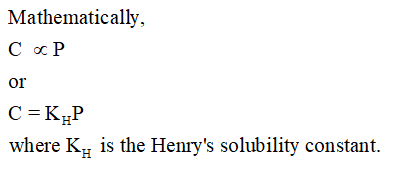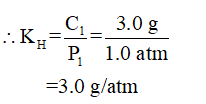# At 0 °C and 1.00 atm, as much as 3 g of O2 can dissolve in 1 L of water. At 0 °C and 1.7 atm, how many grams of O2 dissolve in 1 L of water?

Question
45 views

At 0 °C and 1.00 atm, as much as 3 g of O2 can dissolve in 1 L of water. At 0 °C and 1.7 atm, how many grams of O2 dissolve in 1 L of water?

check_circle

Step 1

At 0 °C and 1.00 atm, as much as 3 g of O2 can dissolve in 1 L of water. At 0 °C and 1.7 atm, grams of O2 dissolved in 1 L of water are to be calculated.

Step 2

Henry’s Law:

It states that the quantity of an ideal gas (C) that dissolves in a definite volume of liquid at a constant temperature is directly proportional to the partial pressure of the gas (P).Step 3

Now, first determine the value of KH for O2.

At 0 °C and 1.00 atm, C1 = 3.0 g

P1 = 1.0 atm...

### Want to see the full answer?

See Solution

#### Want to see this answer and more?

Solutions are written by subject experts who are available 24/7. Questions are typically answered within 1 hour.*

See Solution
*Response times may vary by subject and question.
Tagged in

### Chemistry